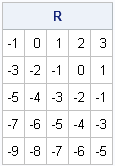In using a vector-matrix language such as SAS/IML, MATLAB, or R, one of the challenges for programmers is learning how to vectorize computations. Often it is not intuitive how to program a computation so that you avoid looping over the rows and columns of a matrix. However, there are a few tricks that tend to come up again and again, and are worth learning so that you can write efficient programs.

The other day I saw a SAS/IML program that constructed a structured matrix. Structured matrices come up often when constructing covariance matrices for time series and mixed models. The following statements are similar to the program I saw. Given a data vector, z, and a parameter, b, the program constructs a matrix R as follows:

```proc iml; n=5; b=2; /* parameters: matrix size and "increment size" */ z = T(1:n); /* the data vector: set to {1,2,...,n} for example */   /* Goal: construct the matrix R from n, b, and z */ R = j(n,n); do i = 1 to n; /* for the i_th row */ do j = 1 to n; /* for the j_th column */ R[i,j] = z[j] - b*z[i]; /* assign R[i,j] */ end; end; print R;```If you only need to construct this matrix once, it doesn't matter that you use a double loop over rows and columns to assign each element of the matrix. However, in general, when you see a loop over rows and columns, you should ask yourself whether the loops can be replaced by vector or matrix computations.

The program that I saw constructed this matrix as part of an iterative algorithm in which the data vector z was changing for each iteration. These double loops were being executed thousands of times. Therefore, it is worthwhile to investigate whether the computation can be vectorized.

### Step 1: Identify constant expressions within a loop

In computer science, a common optimization trick is to "hoist" or "lift" a constant expression outside of a loop so that the expression is evaluated once, rather than for each iteration. The same technique is often the first step in vectorizing a computation. Notice that the inner loop of our example contains the expression b*z[i], which does not depend on j, and therefore can be hoisted out of the inner loop, as follow:

```/* move constant out of inner loop */ R = j(n,n); do i = 1 to n; c = b*z[i]; do j = 1 to n; R[i,j] = z[j] - c; end; end;```

Obviously the result is the same, but how has this helped us? Read on.

### Step 2: Replace an inner loop by a vector operation

The advantage of the new code is that now you can look at the inner loop and recognize it as a vector operation. The inner loop assigns the quantity z` – c to the ith row of R, where z` is the transpose of the column vector, z. Therefore, you can replace the inner loop by a vector operation:

```/* rewrite as row operation with transpose of z */ R = j(n,n); do i = 1 to n; c = b*z[i]; R[i,] = z` - c; end;```

This step alone provides a huge boost to efficiency. Instead of performing n2 scalar operations, the program now performs n vector operations.

### Step 3: Replace a series of vector operations with a matrix operation

To achieve greater efficiency, ask yourself whether a loop that performs a vector operation for each row can be replaced by a matrix operation. In this example, notice that z` does not depend on the iteration variable i, so again we can consider hoisting it out of the loop. The loop uses z` as the starting value for each row of R, before subtracting some quantity. What would happen if we use the SAS/IML REPEAT function to construct a matrix with n rows, where each row is a copy of z`? A moment's thought will convince you that the following matrix expression is equivalent to the original double loop:

```/* matrix-vector computation */ R = repeat(z`,n); /* matrix where each row is z` */ R = R - b*z; /* subtract a quantity the varies for each row */```

The last line (which subtracts a column vector from a matrix) makes sense because the SAS/IML language "knows what you want" and subtracts the ith element of the vector from the ith row of the matrix. Equivalently, you can write this expression as a compact one-liner:

`R = repeat(z`,n) - b*z; /* ultimate simplification */`

So there you have it. A double loop over rows and columns has been rewritten as a single matrix-vector computation. The original program executed the double loop thousands of times (with much larger values of n). Replacing the loops by this matrix expression greatly improves the computational efficiency of the program, and it also eliminates a lot of "clutter" by making the program shorter. If you think that the final result is cryptic, you can add a comment that describes what it does.

If you look at the original loops and then at the final expression, it looks like magic. But if you break down the problem into a series of small vectorizations, the science is evident. Hoisting a constant expression out of a loop is easy. Replacing the inner loop with a vector operation is slightly harder, but provides a great payoff. Making the leap to a matrix operation requires more thought and a knowledge of SAS/IML functions, but the potential gains are worth the effort.

Share